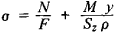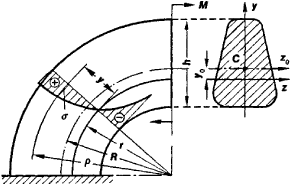# Curved Beam

## curved beam

[¦kərvd ′bēm]
(engineering)
A beam bounded by circular arcs.
McGraw-Hill Dictionary of Scientific & Technical Terms, 6E, Copyright © 2003 by The McGraw-Hill Companies, Inc.
The following article is from The Great Soviet Encyclopedia (1979). It might be outdated or ideologically biased.

## Curved Beam

(in strength of materials and the theory of elasticity), a body whose geometric shape is formed by the motion in space of a plane figure (called the cross section of the curved beam); its center of gravity always follows a certain curve (the axis), and the plane of the figure is normal to the curve. A distinction is made between curved beams with constant cross section (for example, the link of a chain composed of oval or circular rings) and with variable cross section (for example, the hook of a crane) and between plane beams (with a plane axis) and three-dimensional beams (with a three-dimensional axis). A special variety of curved beam is the naturally twisted curved beam, whose plane cross-sectional figure moves along its axis and simultaneously rotates around a tangent to the axis (for example, the blade of an aircraft propeller or fan).

The design of a plane curved beam (Figure 1) with a symmetrical cross section (the axis of symmetry lies in the plane of curvature) taking into account the effect of a load lying in the plane of symmetry consists in the determination of stresses normal to the cross section according to the formulawhere F is the area of the cross section, N is the longitudinal force, M is the bending moment in the cross section defined with respect to the axis Z0 passing through the center of gravity of the cross section (C), y is the distance from the fiber being examined to the neutral axis z, p is the radius of curvature of the fiber being examined, and Sz = Fy0 is the static moment of the cross-sectional area with respect to the axis z. The displacement Y0 of the neutral axis relative to the center of curvature of the curved beam is always directed toward the center of curvature of the curved beam and is usually determined from special tables. For a circular cross section, Y0 ≈ d2/16R; for a rectangular cross section, Yh2/12R (R is the radius of curvature of the axis of the curved beam; d and h are the diameter and height of the cross section of the beam, respectively). Normal stresses in a curved beam have their maximum values (in absolute magnitudes) near the concave edge of a beam and vary in the cross section according to a hyperbolic law. For small curvatures (R > 5h) the determination of normal stresses can be made in the same way as for a straight beam.Figure 1. Distribution of normal stresses in the cross section of a curved beam upon pure bending

### REFERENCE

Beliaev, N. M. Soprotivlenie materialov, 14th ed. Moscow, 1965.

L. V. KASAB’IAN

Mentioned in ?
References in periodicals archive ?
The model allows determining the stress condition of curved beam due bending.
In terms of mechanical properties, many mechanical models of the forming direction, such as the pyramid model , laminated cantilever beam model , small curved beam model , microelement spring model , and porous material model , have been put forward.
Evaluation of Displacements and Stresses in Horizontally Curved Beam, ATLSS Reports, Paper 74, 249 p.
In applying finite element method to the approximate solution of curved beam problems, the following procedure can be considered [28-30]: Curved beam is modeled as a finite element as shown in Figure 1.
The equations for a curved beam are more complicate and sophisticated because of curvilinear geometry.
This curvature is then referred to as the initial curvature, and the system is treated as if a force is acting upon an initially curved beam .
"It is quite a large house, and with the annexe as well, we don't need all this space.'' Because of it's age, the house is full of features of character including some very substantial beams and one curved beam in the hallway which is thought to have come from a ship.
Results of modeling for a straight and a curved beam will be presented and discussed along with the experimental ones.
DQEM analysis of out-of-plane vibration of nonprismatic curved beam structures considering the effect of shear deformation, Advances in Engineering Software 39(6): 466-172.

Site: Follow: Share:
Open / Close